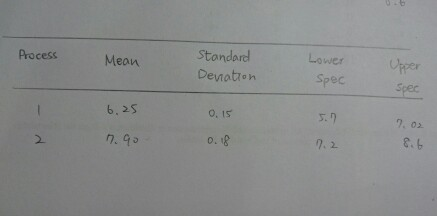# Question & Answer: Process Mean standa Deviation Lower Spec see…

Process Mean standa Deviation Lower Spec see

Determine if the following process are capable.Don't use plagiarized sources. Get Your Custom Essay on
Question & Answer: Process Mean standa Deviation Lower Spec see…
GET AN ESSAY WRITTEN FOR YOU FROM AS LOW AS \$13/PAGE

Process 1

USL = 7.02

LSL = 5.70

Cp = USL – LSL / 6*sigma = (7.02 – 5.70) / (6*0.15) = 1.467

Cpk = min[(USL – mean / 3*sigma), (mean – LSL / 3*sigma)] = min(7.02 – 6.25/3*0.15 , 6.25 – 5.70/3*0.15) = 1.22

All points in 6-sigma limits lie betwwen the USL, LSL range, hence the process is capable.

Process 2

USL = 8.60

LSL = 7.20

Cp = USL – LSL / 6*sigma = (8.60 – 7.20) / (6*0.18) = 1.297

Cpk = min[(USL – mean / 3*sigma), (mean – LSL / 3*sigma)] = min(8.60 – 7.90/3*0.18 , 7.90 – 7.20/3*0.18) = 1.296

All points in 6-sigma limits lie betwwen the USL, LSL range, hence the process is capable, also the process is perfectly centered.